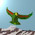## Saturday, December 17, 2011

### The Condensate Scale II

Yesterday we didn't write out the $(W^{+}, W^{-}, Z)$ bosons as a Koide triplet, so let us do that now. This triplet is clearly of the form

$(1,1, \frac{\alpha}{2})$

where $\alpha \cos \theta_{W} = 2$, for the Weinberg angle $\theta_{W}$. The eigenvalue set at the Koide phase $\phi = 0$ is

$\lambda \in \{ 1 + \alpha, 1 - \frac{\alpha}{2} \}$

with multiplicity $2$ for the second eigenvalue. At $\theta_{W} = \pi /6$ we obtain

$\lambda \in \{ 1 + \frac{4}{\sqrt{3}}, 1 - \frac{2}{\sqrt{3}} \}$.

Squaring and summing the rest mass triplet for the Higgs, we obtain $m_{H} = 11/2$ exactly, in the units chosen, which just happen to be the Koide scale for the top quark triplet.

#### 1 comment:

1.So this post is probably junk, because the second triplet is some random triplet of form $(1,1,x)$. But note what happened: the $(W,W,Z)$ triplet is used to write down a real Koide matrix, which itself has a triplet. So one can define a whole sequence of triplets by feeding each set into a (real) Koide matrix. Not sure why each triplet in the sequence should be able to define the Higgs scale, but perhaps $(W,W,Z)$ is not the only one.

Note: Only a member of this blog may post a comment.